## Solving SBT lesson 2 Surrounding area and volume of rectangular box, cube (C3 Math 7 – Horizon) – Math book

Solve SBT lesson 2 Surrounding area and volume of rectangular box, cube (C3 Math 7 – Horizon)
==============

### Solution 1 page 56 SBT Math 7 Creative horizon episode 1 – CTST

Given that each unit block has a volume of 1 cm3. Calculate the volume of the blocks in Figure 4.## Detailed instructions for solving Lesson 1

Solution method

We count the number of unit cubes in each figure.

Detailed explanation

a) Observing Figure 4a), we see that this block is assembled by 12 unit blocks (2 rows, 6 blocks each), so the volume of the block is 12 cm3.

b) Observing Figure 4b), we see that this cube is assembled by 6 units, so the volume of the cube is 6 cm3.

c) Observing Figure 4c), we see that this cube is assembled by 24 units (with 2 columns, each column is 12 blocks), so the volume of the cube is 24 cm3.

–>

— *****

### Solution 2 page 56 SBT Math 7 Creative horizon episode 1 – CTST

A rectangular tank and a bottle of the same size and volume as Figure 5. Show that a full bottle of water is poured into the tank.

a) Calculate the volume of the tank.

b) Calculate the height of the water level after pouring a bottle of water into the tank.

c) If the tank is to be filled, how many bottles of water will be needed.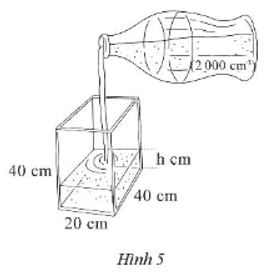## Detailed instructions for solving Lesson 2

Solution method

a) Since the amount of water in the bottle is poured into the tank, it is necessary to add the amount of water in the available tank plus the volume of water in the bottle

b) We use the formula to calculate the volume of a rectangular box divided by the area of ​​the base to get the height

c) We calculate the volume of each bottle and then divide the volume of the tank by the number of bottles that need to be filled to fill the tank.

Detailed explanation

a) The water tank is in the shape of a rectangular box with the lengths of the bottom two sides of 20 cm, 40 cm and a height of 40 cm.

The volume of the tank is: V = 20 . 40 . 40 = 32 000 (cm3).

b) Observe that a water bottle has a volume of 2 000 cm3 So when a full bottle of water is poured into the tank, the volume of water in the tank is 2 000 cm .3.

Therefore, the height of the water level after emptying a bottle of water into the tank is:

h=\(\dfrac{{2000}}{{20.40}} = 2.5\)(cm).

c) If the tank is full, the number of water bottles needed is: 32 000 : 2 000 = 16 (bottles).

–>

— *****

### Solution 3 page 57 SBT Math 7 Creative horizon episode 1 – CTST

A tank is the size of Figure 6. The thickness of the tank on all four sides and bottom is \(\dfrac{1}{4}\) inches.Calculate the volume of the tank.

## Detailed instructions for solving Lesson 3

Solution method

We need to calculate the actual internal volume of the tank, then we need to calculate the length, width, and height of the tank.

Detailed explanation

The width of the tank bed (excluding the tank wall) is: \(6 – \dfrac{1}{4} – \dfrac{1}{4} = \dfrac{{11}}{2}\)(in) ).

The length of the tank (excluding the wall) is: \(12 – \dfrac{1}{4} – \dfrac{1}{4} = \dfrac{{23}}{2}\) (in ).

The height of the tank (excluding the wall) is: \(8 – \dfrac{1}{4} = \dfrac{{31}}{4}\) (in). (because the tank does not have a lid, the height of the tank bed = the height of the tank – the thickness of the bottom of the tank).

The volume of the tank is: V = \(\dfrac{{11}}{2}.\dfrac{{23}}{2}.\dfrac{{31}}{4} = \dfrac{{7843}} {{16}} = 490.1875\) (in3).

–>

— *****

### Solution 4 page 57 SBT Math 7 Creative horizon episode 1 – CTST

A prismatic flask has the bottom dimensions and height as shown in Figure 7. Nam pours some water into it, and then measures the distance from the water level after pouring to the mouth of the jar is 6 cm. How many liters of water are poured in?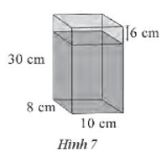## Detailed instructions for solving Lesson 5

Solution method

Calculate:

+ The height of the water level poured into the tank

+ Volume of water level poured into the tank

Detailed explanation

The height of the water level in the tank is: 30 – 6 = 24 (cm).

The volume of the water level poured into the tank is: V = 8 . ten . 24 = 1 920 (cm3).

Exchange: 1 920 (cm3) = 1.92 dm3 = 1.92 liters.

So the number of liters of water poured into the tank is 1.92 liters.

–>

— *****

### Solution 5 page 57 SBT Math 7 Creative horizon episode 1 – CTST

A cargo vehicle has trunk dimensions of 19 ft, 8 ft, and 8 ft (Figure 8) (1 fl ≈ 30.48 cm). One box is 2 ft, 2 ft and 1 ft. The maximum number of crates can be carried by the trunk if you know how to arrange it properly.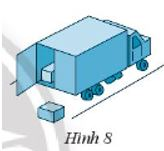## Detailed instructions for solving Lesson 5

Solution method

We calculate the volume of the trunk and then divide by the volume of each box to get the maximum number of boxes that can be stacked.

Detailed explanation

Car and cargo boxes are rectangular in shape.

The volume of the tank is: V = 19 . 8 . 8 = 1 216 (ft3).

The volume of a box is: v = 2 . 2 . 1 = 4 (ft3).

The number of boxes that can be carried if they know how to arrange them properly is: 1 216 : 4 = 304 (cartons).

–>

— *****

### Solve lesson 6 page 57 SBT Math 7 Creative horizon episode 1 – CTST

An aquarium has a bottom size of 1 m, 0.5 m (Figure 9), the permissible water level is 0.4 m. A can has a capacity of 10 liters, how many cans of water to fill to the allowed water level?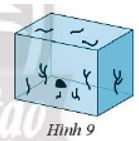## Detailed instructions for solving Lesson 6

Solution method

First we calculate the amount of water allowed to pour into the tank, then divide the volume found by 10 liters to find the number of cans.

Detailed explanation

The volume of the tank with the height from the bottom to the allowable water level is:

V = 1 . 0.5 . 0.4 = 0.2 (m3).

Exchange 0.2 m3 = 200 dm3 = 200 liters.

Therefore, it is necessary to pour 200 liters of water to the allowable water level.

Each can has a capacity of 10 liters.

So the number of cans of water to be poured is: 200 : 10 = 20 (cans)

–>

— *****

### Solve lesson 7 page 57 SBT Math 7 Creative horizon episode 1 – CTST

Nam has 20 cubes of side 4 cm (Figure 10), which must be packed in boxes to be shipped to Khanh. Each box has size 8cm, 10cm, 8cm. How many boxes are needed to close the above 20 cubes?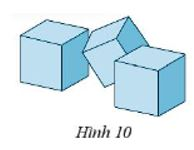## Detailed instructions for solving Lesson 7

Solution method

First we calculate the volume of each block of side 4cm, then we calculate the volume of each box and then see how many blocks each box can hold and multiply to get the number of boxes that need to be closed for 20 blocks.

Detailed explanation

The cube has a side of 4 cm, the dimensions of the container are 8 cm, 10 cm, 8 cm.

Therefore, when placing the cubes in the box, we can only stack up to 2 rows (stacking two cubes on top of each other, the height of the two cubes is 4 . 2 = 8 cm is equal to the height of the box) and Each row is a maximum of 4 blocks, so each box can hold up to 2 . 4 = 8 (cube).

Which 20 : 8 = 2 (remainder 4).

So 3 boxes are needed to hold 20 cubes.

–>

— *****

### Solve problem 8 page 57 SBT Math 7 Creative horizon episode 1 – CTST

Two rectangular boxes are joined together as shown in Figure 11.

a) Calculate the volume of the block.

b) Calculate the total area of ​​the block.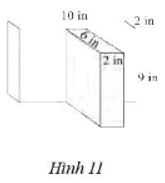## Detailed instructions for solving Lesson 8

Solution method

a) We calculate the volume of each rectangular box. Their sum is the volume of the compound.

The volume of a rectangular box of dimensions a, b, c is: V = abc

b) We calculate the total area of ​​the 2 boxes and then subtract 2 times the area of ​​the tangent parts

The total area of ​​a rectangular box with width a, length b, height c is: \(S = S_{xq}+S_{base}=2.(a+b).c + 2.ab\ )

Detailed explanation

a) The rear rectangular box has dimensions of 10 in, 2 in, 9 in, so the volume is:

DRAWfirst = 10 . 2 . 9 = 180 (in3).

The front rectangular block has dimensions of 6 in, 2 in, 9 in, so the volume is:

DRAW2 = 6 . 2 . 9 = 108 (in3).

Two rectangular blocks combined as shown in Figure 11 have the volume:

V = Vfirst + DRAW2 = 180 + 108 = 288 (in3).

b) The total area of ​​the compound is equal to the sum of the total areas of the two blocks minus twice the area of ​​​​the surfaces that touch each other.

The total area of ​​the rectangular box is equal to the sum of the surrounding area and the area of ​​the two bases.

The total area of ​​the rectangular box behind is:

Sfirst = 2 . (10 + 2) . 9 + 2 . ten . 2 = 256 (in2)

The total area of ​​the front rectangular box is:

S2 = 2 . (6 + 2) . 9 + 2 . 6 . 2 = 168 (in2)

The contact part of the two blocks above is a rectangle with dimensions of 2 in and 9 in.

The total area of ​​the block is:

S = (Sfirst + WILL2) – 2 . 2 . 9 = (256 + 168) – 36 = 388 (in2)

–>

— *****